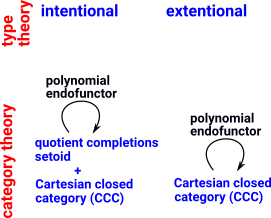# Maths - Type Theory to Category Theory

We can relate type theory to category theory by linking:

• types to objects
• terms to arrows
 So if a category has various objects each of these could be related to a type.### Example of Set

How could a set type be related to a set category?

In the diagram below each object is a set of a given size.

 This diagram doesn't yet reflect the nature of set since set category can have an arrow from every set to every other set except empty set.In order to construct Nat or Vect above we need to call multiple constructors for each instance, for example, to constuct '2' we need to call Z and S and S. So is this a single instance of a type or 3 instances?

## Category Theory, Logic and Type Theory

Category Theory Logic Type Theory
object say A, B ...   type
arrow say m:A->B relation
proved term
inhabited term
idA reflexitiviy
given m:A->B and n:B->C there is n•m:A->C
given e:E->A then idA•e=e
and given m:A->B then m•idA=m

given m:A->B and n:B->C and l:C->D then l•(n•m)=(l•n)•m

### Cartesian Closed Category (CCC)

 In category theory the locally closed Cartesian category is the counterpart of extensional type theory. For intensional type theory we need to add quotient completions - used for modeling extensional type theories into intensional ones.see W-types

The category C is called Cartesian closed if and only if it satisfies the following three properties:

• It has a terminal object.
• Any two objects X and Y of C have a product X ×Y in C.
• Any two objects Y and Z of C have an exponential ZY in C.

Examples specially relevant to type theory:

• In order theory, complete partial orders (cpos) have a natural topology, the Scott topology, whose continuous maps do form a Cartesian closed category (that is, the objects are the cpos, and the morphisms are the Scott continuous maps).
• A Heyting algebra is a Cartesian closed (bounded) lattice. An important example arises from topological spaces. If X is a topological space, then the open sets in X form the objects of a category O(X) for which there is a unique morphism from U to V if U is a subset of V and no morphism otherwise. This poset is a Cartesian closed category: the "product" of U and V is the intersection of U and V and the exponential UV is the interior of U∪(X\V).
• The category of simplicial sets (which are functors X : Δop -> Set) is Cartesian closed.

http://www.mathreference.com/

Where I can, I have put links to Amazon for books that are relevant to the subject, click on the appropriate country flag to get more details of the book or to buy it from them.Computation and Reasoning - This book is about type theory. Although it is very technical it is aimed at computer scientists, so it has more discussion than a book aimed at pure mathematicians. It is especially useful for the coverage of dependant types.